Social QuestionHow good is your mathematical intuition?

Asked by LostInParadise (25864) April 3rd, 2011

There is solid evidence that we are much better at reasoning in context than in the abstract. I have come up with a simple test of this notion. Consider the following problems.

1. Given a small group of numbers, what would be a simple way of testing that no number is more than 5 times greater than any other number, using a minimum amount of calculation.

2. Now let me contextualize it. Suppose you are among a group of people wearing tags giving their ages. How would you go about checking that nobody is more than 5 times as old as anyone else?

Do you find the solution to the second problem to be more obvious? How would you put into words why your test works? I was not able to find a simple way of putting it into words, but I can prove that the method works with a simple algebraic inequality.

3. Given a small group of numbers, how would you go about showing that no number is greater than the square of any other number, using a minimum amount of calculation?

Do you see the relationship between this problem and the previous one? Does it help to contextualize in terms of people’s ages?

Observing members: 0Composing members: 0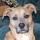As I thought about the solution to the first problem I realized that it’s exactly the way to resolve the second one.

Take the difference between five times the lowest number in the set (5x), and the highest number in the set (X’). If 5x – X’ is a positive number, then you’ve proven the concept.

This is exactly how I’d resolve the second instance. Multiply the age of the youngest person in the room by 5, and see if that’s a larger number than the age of the oldest person. In context it might be simpler, because it’s probably easier to “see” if you have, for example, a college senior as the youngest person in the room (probably at least 20 or 21 years old), then you’d need a 100-year-old-plus to exceed the 5x value. And that might be simple enough to see if no one appears to even be in their 80s.

Problem 3 is a completely different problem, because there you have to work with much smaller numbers. “No number greater than the square of any other number” means you will be working in the range of -1 to 1.

WasCy (10095)“Great Answer” (1) Flag as…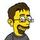The first one I knew without thinking or even putting words to the thoughts.

The second one took me a moment to think about.

The third one is easier than the second.

As for putting it into words…. the best I can manage is min<=max/5. y<=o/5, and s^2>l

The ages make is slightly harder for me, and putting my thought process into words is a bit harder still since I see pictures, not words.

jerv (31034)“Great Answer” (0) Flag as…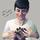When I see something like your second example, my immediate first reaction is to convert the words to numbers, variables, and formulae, as I would do with any word problem. So I honestly treat your first two examples in exactly the same way, except the second one has the added difficulty of having to translate to numbers (not hard in your example, but word problems have the potential to make a usually straight-forward mathematical concept cumbersome).

I would solve the first two examples by either multiplying the smallest number in the group by five and checking if the result if smaller or larger than the largest number in the group, or by dividing the largest number and comparing to the smallest.
In the third example, I was square the smallest number (easier than taking the swaure root of the largest) and see how it compared to the largest.

@WasCy Not so, I don’t think. For example 2 and 3 form a group of numbers >1 neither of which is larger than the square of the other.

Mariah (25831)“Great Answer” (0) Flag as…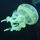Conceptualizing even intuitively multiplying by 5’s is fundamentally easy…don’t even have to think about it especially when applying it to age difference. I know all 10 year olds and younger would qualify compared to me so that is a quite obvious comparison. And I would pay money to see someone still alive at 5 times my age. Moldy oldy.

Cruiser (40401)“Great Answer” (0) Flag as…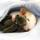In each case, the difficulty depends on how difficult it is to sort the numbers because the calculations involve only the greatest G, the least L, and 5. In the first case: G/L <= 5, and the second, G/L <= L. If you small set is something like { e^pi, pi^e, e^(sqrt(10.2)), ... }, sorting might be the most difficult part of the solution.

The difficulty most people have with “word problems” in elementary algebra suggests that you may not have selected the optimal subject for your experiment because if Jane is twice as old as Sue and….

ratboy (15157)“Great Answer” (0) Flag as…Thank you all. It seems that you have no problem in working just with numbers. The proof that it is only necessary to look at the smallest and largest goes like this:
Let G=greatest and L=least and verify that G<= 5L Then given any X1 and X2,
X2<=G<=5L<=5X1. Working with squares follows the same pattern. I find it interesting that our intuition leads us to the right answer without having to work out the details.

LostInParadise (25864)“Great Answer” (0) Flag as…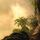In this particular case the first one is so clear that there seems no particular advantage in making a narrative out of it, but I believe there might be instances where everyday intuitions might help or hinder the outcome. Or that a context can provide a quicker solution than plugging in the numbers. Anyone want to try come up with examples of these questions?

lifeflame (5902)“Great Answer” (0) Flag as…@Mariah

You’re right, of course. It was late and I was tired, and the UConn women had just lost their semifinal game against ND, and I read that problem backwards, such that “no number’s square shall be greater than another number in the set”.

WasCy (10095)“Great Answer” (0) Flag as…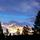@Mariah While I think your counter example expresses what the OP intended to ask, it does not answer the question as it is presented. Reading the third question after the first two makes you assume that you’re only considering numbers in the provided set. However, the question states, “Given a small group of numbers, how would you go about showing that no number is greater than the square of any other number…” When writing mathematics, every word matters. We cannot assume the OP meant “any other number in the set” when that is not what is written.

However, while I think @WasCy is onto something, I disagree with his conclusion. We cannot have a number that is greater than the square of every real number. To demonstrate this, we need only show that there exists one square that is greater than a given number. We begin by considering an arbitrary positive number. If the number is greater than 1, it is less than its own square. If the number is less than 1, it is less than the square of its reciprocal. Obviously, if the number is 1, it is less than the square of any number greater than 1. So we have that every positive number is less than some square. Naturally, if we consider a negative number, it is less than its own square, and 0 is less than 1 squared. Thus we have shown that there does not exist a real number that is greater than the square of any other number.

bobbinhood (5894)“Great Answer” (0) Flag as…@bobbinhood I suppose if the question really meant “no number greater than the square of any other number” you could either interpret that as you did, that is searching for a number that is greater than the squares of all numbers (no such number exists, as you concluded). Another way to interpret those words, I think, is that the numbers in the group are all less than or equal to x^2, where x is any possible real number. In this case the numbers in the group could be less than or equal to zero. But I still think it meant “any other number in the group” even though that’s not exactly what was said. Precise wording is important, otherwise things are up for interpretation.

This is just an example of how word problems can complicate things.

Mariah (25831)“Great Answer” (0) Flag as…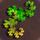In my mind, all three are different forms of the same problem: Given a group of numbers, prove that f(a number x) is never less than a number y. To solve this, I would compare the smallest f(x) to the largest number. In problems 1 and 2, the smallest f(x) is given by the smallest number. If five times the smallest number is greater than the largest number, then five times any number will always be greater than any other number. With problem 3, the smallest f(x) is not given by the smallest number, but the smallest absolute value. Once if find the number with the smalest absolute value, I square it and compare it to the largest number, just like I did in problems 1 and 2.

I think that problem two seemed harder than problems one and three.

PhiNotPi (12670)“Great Answer” (0) Flag as…I can see how presenting something as a word problem may make it more confusing. I was trying to come up with something like the Wason selection problem

LostInParadise (25864)“Great Answer” (0) Flag as…I scrolled down to read the abstract version first and had no trouble with it. It intrigues me that it makes a difference for so many people. 75% success rate versus 5% success rate is a huge difference.

bobbinhood (5894)“Great Answer” (0) Flag as…Actually, I think that the word problem presented second is maybe not ‘only’, but ‘mostly’ simpler to resolve because of the reduced subset of “all numbers” that could be used.

That is, we know that human ages run from 0 to around 120 or so.

The potential universe of “all possible numbers to select from” to satisfy the grouping in the first example is much larger. For example, the “human age” question omits the problem of how to handle negative numbers. Is -25 “five times as large” as -5, or is it five times smaller? When I’m dealing with “ages of people in a room”, it’s not even a consideration.

WasCy (10095)“Great Answer” (0) Flag as…@WasCy But in the abstract question, we only care about the number 3. In the question of the ages, we care about all numbers above 20. By your reasoning, doesn’t that make the abstract case similar? Or do I misunderstand?

bobbinhood (5894)“Great Answer” (0) Flag as…